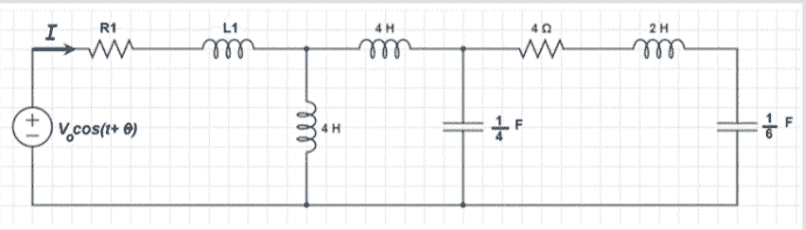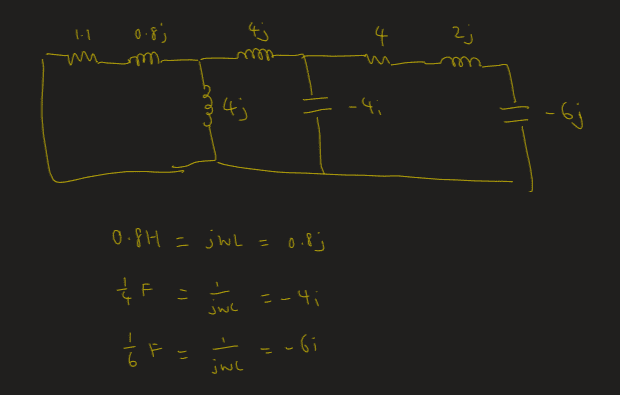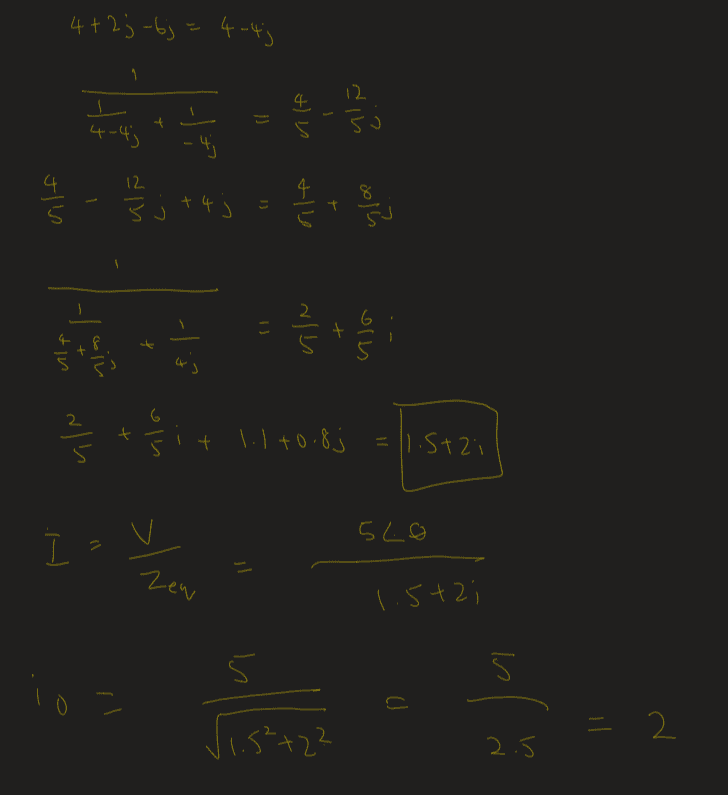# Circuits - AC circuits / phasor

Homework Statement:
Given the following circuit with the voltage source V=5 cos(t+θ) and the resistor R1=1.1(Ω) and the inductor L1=0.8(H). The impedance Z_eq of the circuit and the phasor current I can be respectively described as

$$Z_{eq} =a+jb$$
$$I= I_o ∠ Φ$$

Find a, b, and Io in these expressions.
Relevant Equations:
-Hi, my school has no answer key provided. and would like to check if this is correct. Thanks!

gneill
Mentor
Hi. It looks like you've correctly found the equivalent impedance and the magnitude of the current (both without units though). However, you'll need to account for the phase angle θ of the source in your Io.

Hi. It looks like you've correctly found the equivalent impedance and the magnitude of the current (both without units though). However, you'll need to account for the phase angle θ of the source in your Io.
From the question, I_o is without phase angle. where I = I_o ∠ Φ. That is why i didn't account for the phase angle.

side note: how do you input latex for like I_o in the text

It's ##I_{0}## [sharp(#) sharp(#) I_{0} sharp(#) sharp(#) ]

gneill
Mentor
From the question, I_o is without phase angle. where I = I_o ∠ Φ. That is why i didn't account for the phase angle.
Presumably you'll need to specify what ##\Phi## is, no?

Presumably you'll need to specify what ##\Phi## is, no?
i dont need to but is it even possible, since they did not give angle ##\theta##

You need ##\theta## to compute ##\varphi##

gneill
Mentor
i dont need to but is it even possible, since they did not give angle ##\theta##
You can specify ##\Phi## in terms of ##\theta## and the impedance's angle.

•DaveE
DaveE